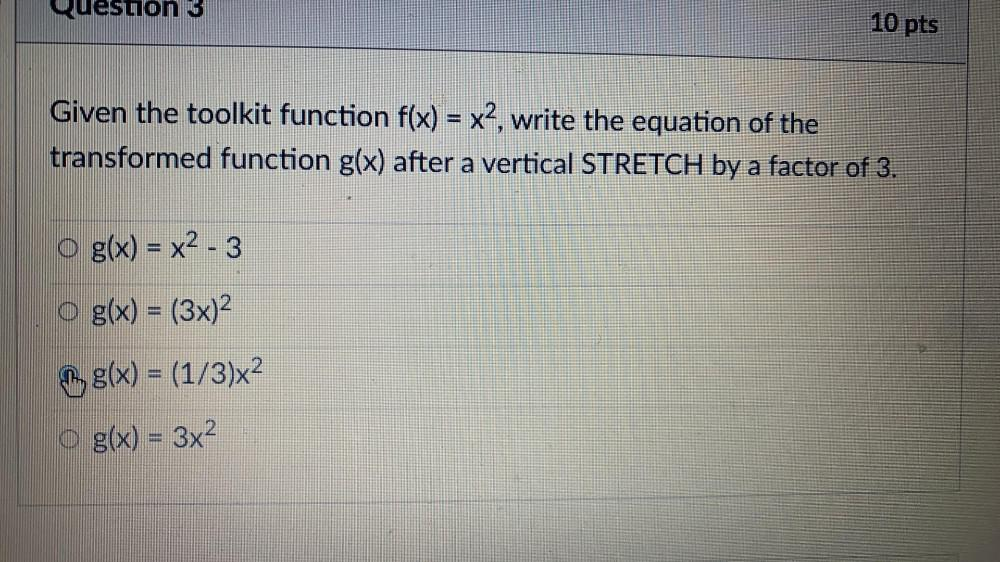Question:

# Quesuon 3 10 pts Given the toolkit function f(x) = x2, write the equation of the transformed function g(x) after a vertical STREQuesuon 3 10 pts Given the toolkit function f(x) = x2, write the equation of the transformed function g(x) after a vertical STRETCH by a factor of 3. g(x) = x2 - 3 o g(x) = (3x)2 , g(x) = (1/3)x2 O g(x) = 3x2 S